Courses

# Test: Gas Turbine - 1

## 20 Questions MCQ Test RRB JE for Mechanical Engineering | Test: Gas Turbine - 1

Description
This mock test of Test: Gas Turbine - 1 for Mechanical Engineering helps you for every Mechanical Engineering entrance exam. This contains 20 Multiple Choice Questions for Mechanical Engineering Test: Gas Turbine - 1 (mcq) to study with solutions a complete question bank. The solved questions answers in this Test: Gas Turbine - 1 quiz give you a good mix of easy questions and tough questions. Mechanical Engineering students definitely take this Test: Gas Turbine - 1 exercise for a better result in the exam. You can find other Test: Gas Turbine - 1 extra questions, long questions & short questions for Mechanical Engineering on EduRev as well by searching above.
QUESTION: 1

Solution:
QUESTION: 2

### Isentropic compression of saturated vapour of all superheated vapour.

Solution: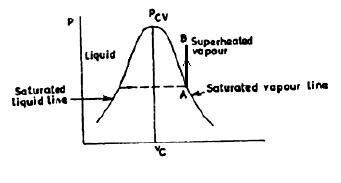QUESTION: 3

### . A gas turbine cycle with heat exchanger and reheating improves

Solution:
QUESTION: 4

Which one of the following is correct?

Solution:
QUESTION: 5

Consider the following statements:

1. The speed of rotation of the moving elements of gas turbines is muchhigher than those of steam turbines

2. Gas turbine plants are heavier and larger in size than steam turbineplants

3. Gas turbines require 'cooling water for its operations

4. Almost any kind of fuel can be used with gas turbines

Which of the statements given above are correct?

Solution:
QUESTION: 6

Assertion (A): The thermal efficiency of a gas turbine plant is low as compared tothat of reciprocating IC engines.

Reason (R): In a gas turbine plant, the maximum pressure and temperature arelow when compared to those of reciprocating IC engines.

Solution:
QUESTION: 7

Consider the following statements pertaining to gas turbines:

1. The degree of reaction of a reaction turbine is the ratio of energytransfer in fixed blade to the overall energy transfer across a stage.

2. The overall pressure drop in a turbine is the product of pressure dropper stage and number of stages.

3. Gas turbine cycle (Brayton cycle) is not as efficient as Rankine cycle forsteam.

Which of the above statements is/are correct?

Solution:
QUESTION: 8

Assertion (A): The thermal efficiency of gas turbine plants is higher compared todiesel plants.

Reason (R): The mechanical efficiency of gas turbines is higher compared to dieselengines.

Solution:

A is false and R is true

QUESTION: 9

Assertion (A): Gas turbines use very high air fuel ratio.

Reason (R): The allowable maximum temperature at the turbine inlet is limited by available material considerations.

Solution:

Though A and R are true, but R is not correct reason for A.

QUESTION: 10

Consider the following statements comparing I.C. engines and gasturbines:

1.     Gas turbines are simple, compact and light in weight.

2.     Complete expansion of working substance is possible in I.C. engines and not in gas turbines.

3.     There is flexibility in the design of different components of gas turbines as different processes take place in different components.

4.     Even low grade fuels can be burnt in gas turbines.Of these statements

Solution:

Low grade fuel can't be burnt in gas turbine since it leads to poor life of turbine blades, etc.

QUESTION: 11

The power coefficient (cp)variation of a wind mill with the speed ratio is correctly shown by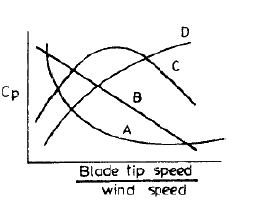Solution:
QUESTION: 12

A Bell-Coleman air refrigeration cycle works as a reversed:

Solution:
QUESTION: 13

Assertion (A): The thermal efficiency of Brayton cycle with regeneration decreases as the compressor ratio increases.

Reason (R): As the   compression   ratio    of   compressor  increases, the   range    oftemperature in the regenerator decreases and the amount of heat recovered reduces.

Solution: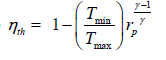QUESTION: 14

A gas turbine works on which one of the following cycles?

Solution:
QUESTION: 15

Which one of the thermodynamic cycles shown in the following figures represents that of Brayton cycle?

Solution:
QUESTION: 16

For a Brayton cycle, match the following using codes given below: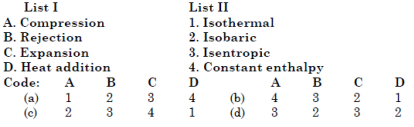Solution:
QUESTION: 17

Use of maximum pressure ratio, corresponding to maximum to minimum cycle temperature ratio in case of Joule cycle gives which one of the following?

Solution:
QUESTION: 18

Consider the T-s diagram shown in thefollowing figure:Actual compression process in gasturbines is indicated by the process.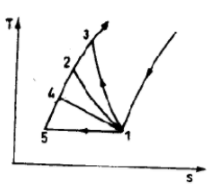Solution:

Curve 1-3 is actual compression process in gas turbine.

QUESTION: 19

The given figure shows four plots A, B, C and D of thermal efficiency against pressure ratio: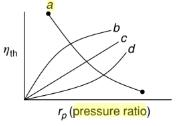The curve which represents that of a gas turbine plant using Brayton cycle (without regeneration) is the one labelled

Solution:
QUESTION: 20

Consider the following statements in respect of gas turbines:

1. Supersonic flow leads to decrease in efficiency.

2. Supersonic flow leads to decrease in flow rate.Which of the statements given above is/are correct?

Solution: